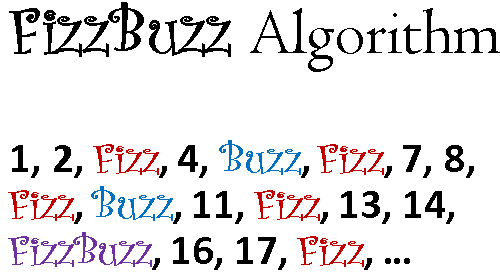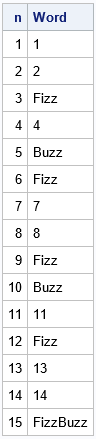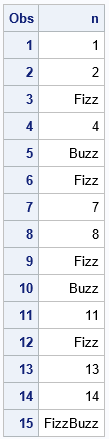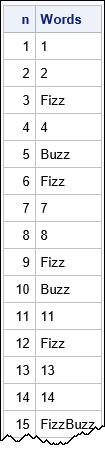Recently, I learned about an elementary programming assignment called the FizzBuzz program. Some companies use this assignment for the first round of interviews with potential programmers. A competent programmer can write FizzBuzz in 5-10 minutes, which leaves plenty of time to discuss other topics. If an applicant can't complete the FizzBuzz program in a required language, the interviewer concludes that they are a weak programmer in that language.

When I heard about the FizzBuzz program, I quickly implemented it in the SAS DATA step. However, it occurred to me that I could think of additional techniques to solve the problem in SAS. Each technique demonstrates different skills and could help an interviewer distinguish between junior-level, intermediate-level, and senior-level SAS programmers. This article introduces the FizzBuzz program for SAS programmers and solves it in the following ways:

• Junior level: Use the SAS DATA step to transform a set of input data
• Intermediate level: Use a function that is defined by using PROC FCMP
• Senior level: Create a user-defined format by using PROC FORMAT
• Statistical level: Write a vectorized SAS IML program### What is the FizzBuzz algorithm?

The FizzBuzz program is presented on the Rosetta Code website. The Rosetta Code site shows the same program written in hundreds of different programming languages, which makes it a convenient way to compare languages. The description of the FizzBuzz program on the Rosetta Code page is as follows:

Write a program that prints the integers from 1 to 100 (inclusive). But:

• for multiples of three, print "Fizz" (instead of the number)
• for multiples of five, print "Buzz" (instead of the number)
• for multiples of both three and five, print "FizzBuzz" (instead of the number)

If you would like to take a minute to implement the program in SAS (or another language!), do so now. A solution is presented in the next section.

### The modified FizzBuzz program in SAS

First, let's slightly adapt the assignment for the SAS programmer. The solution given on the Rosetta Code site uses a DO loop to generate the numbers and the PUT statement to write the result to the log, which is a fine implementation. However, the ability to read and transform existing data is an essential part of SAS programming. Consequently, a better assignment for a SAS programmer would start with a data set of values. The programmer must read the values (whatever they are) and apply the FizzBuzz algorithm to create a new variable in a new data set.

In theory, the input data could be any numerical values, but to stay faithful to the original assignment, you can ask the programmer to create an input data set (Have) that contains the integers 1-100, one per row:

```data Have; do n=1 to 100; output; end; run;```

### A junior SAS programmer writes the FizzBuzz program

Ready to write the FizzBuzz program? A junior-level Base SAS programmer would probably write the following DATA step, which reads the Have data and creates a new 8-character variable named Word that contains either "Fizz," "Buzz," "FizzBuzz," or uses the PUT function to convert the number to a character representation:

```/* Junior programmer */ data Want; length Word \$8; set Have; if mod(n,15)=0 then Word = "FizzBuzz"; else if mod(n,5) =0 then Word = "Buzz"; else if mod(n,3) =0 then Word = "Fizz"; else Word = put(n, 8.); run;   proc print data=Want(obs=15) noobs; var n Word; run;```This is a fine solution. It enables the interviewer to ask about the LENGTH statement, the w.d format, and integer division by using the MOD function. If a programmer omits the LENGTH statement, that indicates a lack of knowledge about character variables in SAS.

Another possibility is that a junior-level programmer could use PROC SQL to write the FizzBuzz program. There is an SQL version of the program at Rosetta Code, and I invite a reader to add the PROC SQL version in a comment to this article.

### An intermediate SAS programmer writes the FizzBuzz program

An intermediate-level programmer understands the power of encapsulation. If the FizzBuzz functionality needs to be used several times, can you encapsulate the program into a reusable function?

In SAS, you can use PROC FCMP to define your own library of useful functions. The documentation for PROC FCMP provides the details and several examples. For this exercise, the key is to have the function return a character value, which means you need to specify a dollar sign (\$) after the argument list (and optionally specify the length). You also need to use the OUTLIB= option to specify the name of the data set where the function is stored. Lastly, you should use the global CMPLIB= option to make the function known to a DATA step.

```/* Intermediate programmer: Use PROC FCMP to define the FizzBuzz function */ /* https://go.documentation.sas.com/doc/en/pgmsascdc/9.4_3.5/proc/n1eyyzaux0ze5ln1k03gl338avbj.htm */ proc fcmp outlib=work.functions.NterView; function FizzBuzz(n) \$ 8; length Word \$8; if mod(n,15)=0 then Word = "FizzBuzz"; else if mod(n,5) =0 then Word = "Buzz"; else if mod(n,3) =0 then Word = "Fizz"; else Word = put(n, 8.); return(Word); endsub; run;   options cmplib=(work.functions); /* make the function available to DATA step */ data Want; length Word \$8; set Have; Word = FizzBuzz(n); run;   proc print data=Want(obs=15) noobs; var n Word; run;```

The output is the same as was shown previously.

### A senior SAS programmer writes the FizzBuzz program

A senior-level programmer understands the power of SAS formats and can create a user-defined format to prevent the wasteful work copying of data. Consider the result of the previous intermediate-level program. The entire Have data set is copied merely to add a new eight-character variable. Think about the wastefulness of this approach if the input data set is many gigabytes in size!

One alternative to copying the data is to create a user-defined format that will format a variable in place without recoding it. Senior-level programmers should be able to explain why using PROC FORMAT is better than copying and recoding variables.

Creating a user-defined format uses the FizzBuzz function that we defined by using PROC FCMP. The documentation of PROC FORMAT has an example that shows how to use a user-defined function to define a custom format. The following program shows how to use the FizzBuzz function to define a custom format in PROC FORMAT:

```/* Senior programmer: Create a format by using the FCMP function */ /* We don't need a new data set with a new variable. Just apply a format to the existing data! */ proc format; value FBFMT other=[FizzBuzz()]; run;   /* use the format */ proc print data=Have(obs=15); format n FBfmt.; run;```This solution is very short because it builds on the previous solutions. It can lead to discussions about efficiency.

### A SAS statistical programmer writes the FizzBuzz program

Advanced statistical programmers use the high-level SAS IML matrix language to program custom analyses. In a matrix language, the ability to vectorize a computation is important. Vectorization means treating data as vector and matrix objects and using vector operations rather than loops to interact with the data. After you read the data into a vector, you can construct binary (0/1) vectors that indicate whether each row is divisible by 3, by 5, or by both. You can then use the LOC function to identify the rows that satisfy each condition, as follows:

```/* SAS IML programmer: Vectorize the FizzBuzz algorithm */ proc iml; use Have; read all var "n"; close; F = (mod(n,3)=0); /* binary variable: is n divisible by 3? */ B = (mod(n,5)=0); /* binary variable: is n divisible by 5? */ FB = F & B; /* binary variable: is n divisible by 3 & 5? */ Words = char(n, 8); /* default: convert the number into a string */ Words[loc(F)] = "Fizz"; /* write to the "div by 3" indices */ Words[loc(B)] = "Buzz"; /* write to the "div by 5" indices */ Words[loc(FB)] = "FizzBuzz";/* write to the "div by 3 & 5" indices */ print n Words;```This program can lead to discussions about efficiency, vectorization, and logical operators on vectors.

### Discussion

The FizzBuzz program assignment is more than a programming exercise. It can provide opportunities for discussing related SAS programming topics. For example:

• Does the implementation handle missing values?
• Does the program correctly handle negative integers? What about 0?
• What does the program do if the input data are not integers? For example, what is FizzBuzz(3.2)?
• How would you modify the program to detect whether the input is not a positive integer and write "Jazz" in that case?
• Suppose the input data set contains one billion observations. Discuss the efficiency of your implementation of FizzBuzz.

### Summary

The FizzBuzz algorithm is an elementary programming assignment that tests whether a programmer has minimal knowledge of a language. It is sometimes used in job interviews to assess the candidate's skills. This article presents a SAS-specific variation on the classic FizzBuzz assignment. It also shows how this elementary problem can be solved by using more sophisticated methods in SAS, such as user-defined functions, user-defined formats, and matrix programming in the SAS IML language. Although the methods might be too difficult for some candidates to write during an interview, a discussion of the enhancements can help assess the candidate's knowledge of advanced techniques in SAS.

In early 2023, many programmers have been impressed by the ability of ChatGPT and Bing Chat to write elementary computer programs. Can an AI chatbot replace a junior-level SAS programmer? In my next blog post, I investigate what you get if you ask Bing Chat to implement the FizzBuzz algorithm in SAS.

The post An interview question for SAS programmers appeared first on The DO Loop.